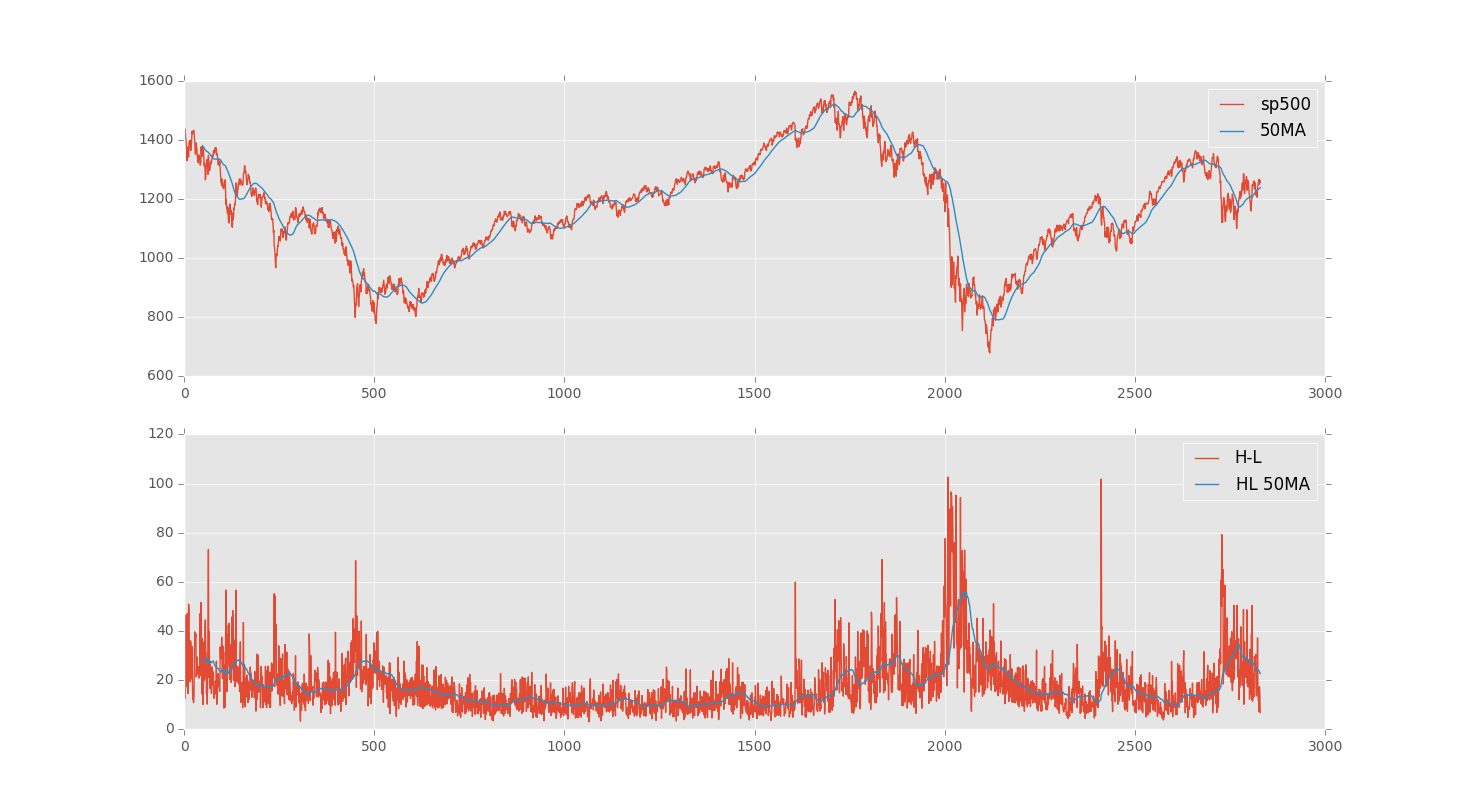## Pandas Basics

Code written in Python 2, but you can either comment out all print statements, or convert them to functions and it will work fine.

```import datetime
import pandas as pd
import pandas.io.data
from pandas import DataFrame
import matplotlib.pyplot as plt
from matplotlib import style

style.use('ggplot')

## grabbing from yahoo finance ###
sp500 = pd.io.data.get_data_yahoo('%5EGSPC',
start=datetime.datetime(2000, 10, 1),
end=datetime.datetime(2012, 1, 1))

# saving to a csv #
sp500.to_csv('sp500_ohlc.csv')

# reading from the csv #

print df.index

# again, just accessing a column.... #

ts = df['Close'][-10:]
print ts

# or a couple:

# can also create new columns:
df['H-L'] = df.High - df.Low

# can also delete:
del df['H-L']

#So besides High minus low, people like to see moving averages:
ma = pd.rolling_mean(close, 50)
print ma[-10:]

#so then let's bring back the high minus low, since ppl like to use that
#sometimes.
# can also create new columns:
df['H-L'] = df.High - df.Low

HLMA = pd.rolling_mean(df['H-L'], 50)

ax1 = plt.subplot(2, 1, 1)
ax1.plot(close, label='sp500')
ax1.plot(ma, label='50MA')
plt.legend()

ax2 = plt.subplot(2, 1, 2, sharex = ax1)
ax2.plot(df['H-L'], label='H-L')
ax2.plot(HLMA, label='HL 50MA')

plt.legend()
plt.show()

```

The next tutorial: